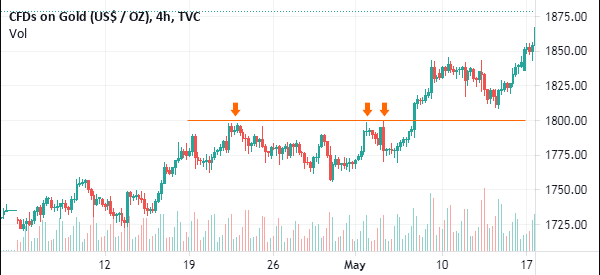# Stock Charts Detection Using Image Classification Model ResNet

## Intro

This tutorial explores image classification in PyTorch using state-of-the-art computer vision models. The dataset used in this tutorial will have 3 classes that are very imbalanced. So, we will explore augmentation as a solution to the imbalance problem.

Data used in this notebook can be found at https://www.nbshare.io/blog/datasets/

## Contents:

• Train-test splitting
• Augmentation
• Creating Datasets
• Random Weighted Sampling and DataLoaders
2. CNN building and fine-tuning ResNet
• CNN
• ResNet
3. Setup and training
4. Evaluation
5. Testing

In :
import os
import random
import numpy as np
import pandas as pd
from PIL import Image
from sklearn.metrics import accuracy_score
from sklearn.model_selection import train_test_split
import torch
from torch import nn
import torch.nn.functional as F
from torch.utils.data import Dataset, DataLoader, WeightedRandomSampler
from torchvision import datasets, models
from torchvision import transforms
import matplotlib.pyplot as plt


Setting the device to make use of the GPU.

In :
device = torch.device("cuda" if torch.cuda.is_available() else "cpu")
device

Out:
device(type='cuda')

Identifying the data paths.

In :
data_dir = "images/"
labels_file = "images_labeled.csv"


Since the labels are in a CSV file, we use pandas to read the file and load it into a DataFrame

In :
labels_df = pd.read_csv(labels_file)

Out:
Image Name Category
0 0Ou5bdH5c094eTqk.jpg Others
1 15i__Nqs70zFkb_h.jpg Others
2 1B7Kf3yXIchfrliL.jpg Others
3 1tKvOK_m3ZEInWe1.jpg Others
4 32d0brxK_-4Ha_Ff.jpg Others

As shown, we have 3 classes that are imbalanced.

In :
labels_df["Category"].value_counts()

Out:
Technical    911
Others       488
News         101
Name: Category, dtype: int64

Creating numerical IDs for each class. The following list and dictionary are used for converting back and forth between labels and IDs.

In :
id2label = ["Technical", "Others", "News"]
label2id = {cl:idx for idx, cl in enumerate(id2label)}


### Train-test Splitting

We use pandas to split the data into an 80-20 split.

In :
train_labels_df, test_labels_df = train_test_split(labels_df, test_size = 0.2)

In :
train_image_names = list(train_labels_df["Image Name"])
train_image_labels = list(train_labels_df["Category"])
test_image_names =  list(test_labels_df["Image Name"])
test_image_labels =  list(test_labels_df["Category"])

In :
train_image_names[:5]

Out:
['FFnZNgvXMAgUuS9.jpg',
'FFiiKZRWQAAsW1X.jpg',
'FFiLBOtUUAUsHV3.jpg',
'FFYyzfpVQAIaN7F.png',
'FFmhojdWUAYRGWT.jpg']
In :
print("Train set size:", len(train_labels_df),
"\nTest set size:", len (test_labels_df))

Train set size: 1200
Test set size: 300


### Augmentation

The solution we follow in this tutorial for data imbalance is to create a random weighted sampler that, in each batch, takes approximately the same number of images from each class. It does so by using replacement sampling with the inferior classes.

However, that alone is not enough. Since there will be replacement in sampling (meaning that the same image can repear twice in a batch), we need to perform augmentation on all images to add some differences.

This is performed using PyTorch "transforms".

For both training and test sets, we will apply the following transformations to create augmented versions of the images:

In :
transform_dict = {'train': transforms.Compose([
transforms.RandomResizedCrop(224),
transforms.RandomHorizontalFlip(),
transforms.ToTensor(),
transforms.Normalize([0.485, 0.456, 0.406], [0.229, 0.224, 0.225])
]),
'test': transforms.Compose([
transforms.Resize((224, 224)),
transforms.CenterCrop(224),
transforms.ToTensor(),
transforms.Normalize([0.485, 0.456, 0.406], [0.229, 0.224, 0.225])
]),}


### Creating Datasets

In :
class ImageDS(Dataset):
def __init__(self, data_dir, image_names, labels, transformations):
self.image_names = image_names
self.labels = [label2id[label] for label in labels]
self.transforms = transformations
self.data_dir = data_dir
self.img_paths = [os.path.join(self.data_dir, name)
for name in self.image_names]

def __len__(self):
return len(self.img_paths)

def __getitem__(self, idx):
"""
Opens an image and applies the transforms.
Since in the dataset some images are PNG and others are JPG,
we create an RGB image (no alpha channel) for consistency.
"""
img = Image.open(self.img_paths[idx])
label = self.labels[idx]
rgbimg = Image.new("RGB", img.size)
rgbimg.paste(img)
rgbimg = self.transforms(rgbimg)

return rgbimg, label


Initializing the Datasets

In :
train_ds = ImageDS(data_dir, train_image_names, train_image_labels, transform_dict['train'])
test_ds = ImageDS(data_dir, test_image_names, test_image_labels, transform_dict['test'])


Plotting an image to verify the changes. As shown, the image is cropped into a 224x224 square as intended.

In :
plt.imshow(train_ds.permute(1, 2, 0))

Clipping input data to the valid range for imshow with RGB data ([0..1] for floats or [0..255] for integers).

Out:
<matplotlib.image.AxesImage at 0x7f00326f6b50>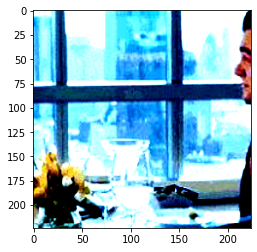The corresponding label:

In :
id2label[train_ds]

Out:
'Others'

PyTorch provides an implementation for random weighted sampling using this class:

WeightedRandomSampler()

This class takes 2 parameters to create the sampler: the weights of each instance of each class, and the size of the dataset. We calculate the weights and create the sampler using this function:

In :
def create_weighted_sampler(ds):
class_prob_dist = 1. / np.array(
[len(np.where(np.array(ds.labels) == l)) for l in np.unique(ds.labels)])
classes = np.unique(ds.labels)
class2weight = {cl:class_prob_dist[idx] for idx, cl in enumerate(classes)}
weights = [class2weight[l] for l in ds.labels]
return WeightedRandomSampler(weights, len(ds))


Initializing samplers:

In :
train_sampler = create_weighted_sampler(train_ds)
test_sampler = create_weighted_sampler(test_ds)


Finally, we use those samplers while creating the DataLoaders. That way the DataLoaders are ready to provide balanced data.

In :
train_dl = DataLoader(train_ds, batch_size=16, sampler = train_sampler)

In :
dataloaders = {"train": train_dl, "test": test_dl}


## CNN building and fine-tuning ResNet

### CNN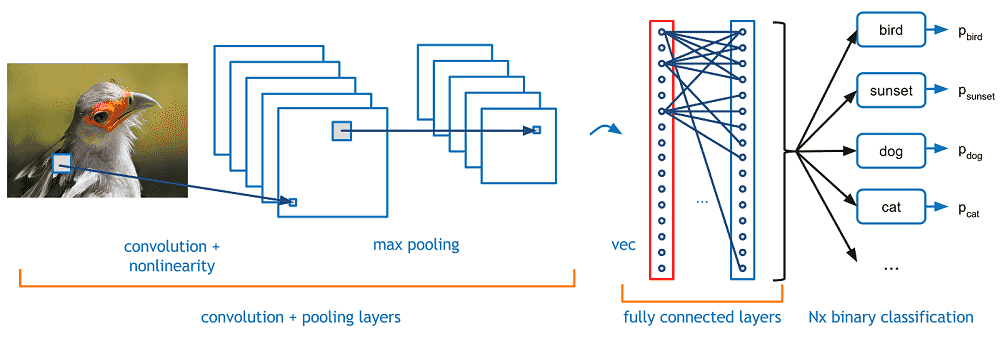The following is a simple CNN model. We use ResNet as the main model in this tutorial, but you can use the CNN below instead by initializing the model to CNN().

In :
class CNN(nn.Module):
def __init__(self):
super().__init__()
self.conv1 = nn.Conv2d(3, 6, 5)
self.pool = nn.MaxPool2d(2, 2)
self.conv2 = nn.Conv2d(6, 16, 5)
self.fc1 = nn.Linear(44944, 120)
self.fc2 = nn.Linear(120, 84)
self.fc3 = nn.Linear(84, 10)

def forward(self, x):
x = self.pool(F.relu(self.conv1(x)))
x = self.pool(F.relu(self.conv2(x)))
x = torch.flatten(x, 1) # flatten all dimensions except batch
x = F.relu(self.fc1(x))
x = F.relu(self.fc2(x))
x = self.fc3(x)
return x


To choose the CNN, run this cell and not the one below it:

In [ ]:
model = CNN()
model = model.to(device)
model

Out[ ]:
CNN(
(conv1): Conv2d(3, 6, kernel_size=(5, 5), stride=(1, 1))
(pool): MaxPool2d(kernel_size=2, stride=2, padding=0, dilation=1, ceil_mode=False)
(conv2): Conv2d(6, 16, kernel_size=(5, 5), stride=(1, 1))
(fc1): Linear(in_features=44944, out_features=120, bias=True)
(fc2): Linear(in_features=120, out_features=84, bias=True)
(fc3): Linear(in_features=84, out_features=10, bias=True)
)

### ResNet

Here, we use ResNet-101 as the model:

In :
model = models.resnet101(pretrained=True)
num_ftrs = model.fc.in_features
# for param in model.parameters(): # Uncomment these 2 lines to freeze the model except for the FC layers.
model.fc = nn.Linear(num_ftrs, 3)

Downloading: "https://download.pytorch.org/models/resnet101-63fe2227.pth" to /root/.cache/torch/hub/checkpoints/resnet101-63fe2227.pth


Sending model to device

In :
model = model.to(device)


Initializing the criterion and optimizer:

In :
criterion = nn.CrossEntropyLoss()
optim = torch.optim.Adam(model.parameters(), lr = 1e-3)


## Setup and Training

In [ ]:
training_losses = []
test_losses = []
for epoch in range(15):  # loop over the datasets multiple times
for phase in ["train", "test"]: # loop over train and test sets separately
if phase == 'train':
model.train()  # Set model to training mode
else:
model.eval()   # Set model to evaluate mode
running_loss = 0.0
for i, data in enumerate(dataloaders[phase], 0): # loop over dataset
# get the inputs; data is a list of [inputs, labels]
inputs, labels = data

labels = labels.to(device)
# forward + backward + optimize
outputs = model(inputs)
loss = criterion(outputs, labels)
_, preds = torch.max(outputs, 1)
loss.backward()

# Performing gradient clipping to control our weights
optim.step()
if phase == 'train':
training_losses.append(loss.item())
else:
test_losses.append(loss.item())
# print statistics
running_loss += loss.item()
print_freq = 10
if i % print_freq == 0:    # print every 10 mini-batches
print('%s: [%d, %5d] loss: %.3f' %
(phase, epoch + 1, i + 1, running_loss / print_freq))
running_loss = 0.0

print('Finished Training')


### Evaluation

In :
plt.plot(training_losses)

Out:
[<matplotlib.lines.Line2D at 0x7f0019ce1090>]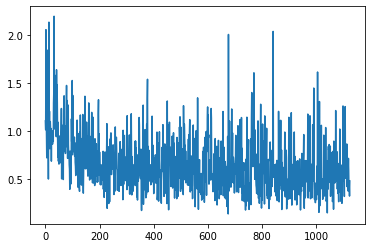In :
plt.plot(test_losses)
plt.ylim([0, 3])
plt.show()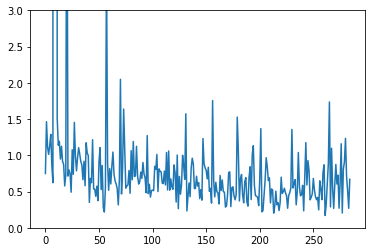We can observe from the training and the losses that the model learned, although it was noisy.

We find the accuracy by predicting the test set:

In :
preds_total = []
for i, data in enumerate(test_dl, 0):
# get the inputs; data is a list of [inputs, labels]
inputs, labels = data

inputs = inputs.to(device)
labels = labels.to(device)

# obtaining predictions
logits = model(inputs)
preds = torch.argmax(logits, 1)
print(i)
preds_total += preds.to('cpu').tolist()

0
1
2
3
4
5

/usr/local/lib/python3.7/dist-packages/PIL/Image.py:960: UserWarning: Palette images with Transparency expressed in bytes should be converted to RGBA images
"Palette images with Transparency expressed in bytes should be "

6
7
8
9
10
11
12
13
14
15
16
17
18

In :
print(type(preds_total), len(preds_total))
print(type(test_ds.labels), len(test_ds.labels))

<class 'list'> 300
<class 'list'> 300

In :
accuracy_score(preds_total, test_ds.labels)

Out:
0.4533333333333333

The accuracy is ~45%

Despite using a SOTA model, advanced image processing, and good imbalance solutions, the accuracy of this 3 class task is relatively low. There are 2 main problems we can observe:

1. There are many incorrect labels in the data. This adds noise in the learning process and confuses the model, preventing it to learn from many instances. The graphs of the loss demonstrate this problem, where the plot increases and decreases sharply. The solution is to recheck the labels.

2. The 2nd problem I observe is the content of the "Other" class. It is always better to avoid including an "other" class in image classification, or at least to keep the instances in the "other" class relatively similar. The "other" images in the data are very random, making it difficult to detect. The solution is to either try training without this class, or to improve the quality of the images in this class. That way, the model is not very confused about the content of this class.

### Testing

To further validate the perforamance, we predict the labels for random images in the test set:

In :
# Get a random test image
random_id = random.randint(0, len(test_labels_df))
img_name, lbl = test_labels_df.iloc[random_id]

In :
img_name, lbl

Out:
('FFdPSh3XsAImGWs.jpg', 'Others')
In :
img = Image.open(os.path.join(data_dir, img_name))
rgbimg = Image.new("RGB", img.size)
rgbimg.paste(img)
img = transform_dict['test'](rgbimg)
plt.imshow(img.permute(1,2,0))

Clipping input data to the valid range for imshow with RGB data ([0..1] for floats or [0..255] for integers).

Out:
<matplotlib.image.AxesImage at 0x7f0019a56c90>In :
# First, send the image to device
img = img.to(device)

In :
# Feed the image to the model
logits = model(img[None, ...])

In :
# Get the class with the highest score
_, preds = torch.max(logits, 1)
pred = preds.item()
id2label[pred]

Out:
'Others'
In :
pred == label2id[lbl]

Out:
True

However, the model is correct for the shown example above, as it predicted category "Others" because it is neither News nor stock chart.